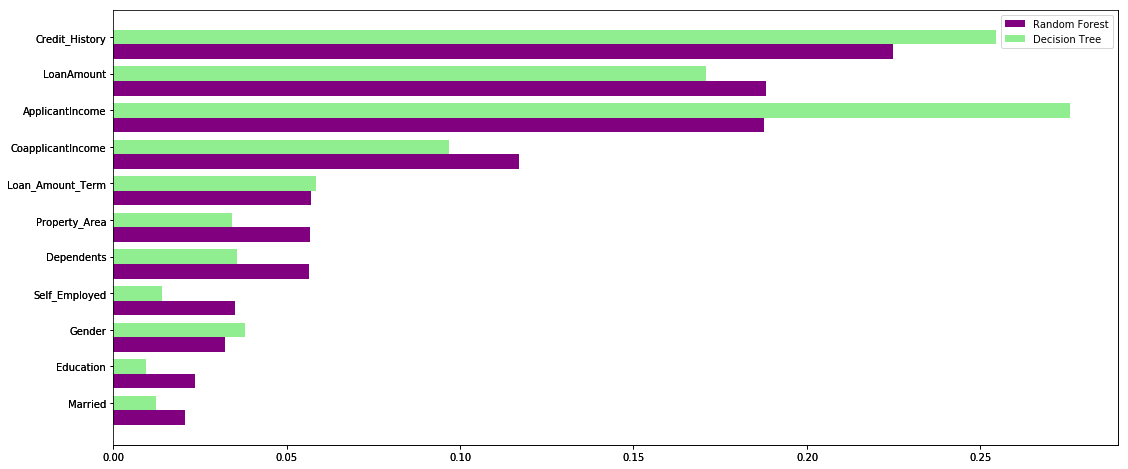• +91-9872993883
• +91-8283824812
• info@ris-ai.com

# Loan Eligibility Prediction For Customer ¶

### Loan prediction for customers using decision tree model and random forest model for comparison. ¶

Let’s start by importing the required Python libraries and our dataset.

In :
import pandas as pd
import numpy as np
import matplotlib.pyplot as plt
from sklearn.metrics import f1_score
from sklearn.model_selection import train_test_split


## Importing the dataset ¶

The dataset consists of 614 rows and 13 features, including credit history, marital status, loan amount, and gender. Here, the target variable is Loan_Status, which indicates whether a person should be given a loan or not.

In :
# Importing dataset

Out:
Loan_ID Gender Married Dependents Education Self_Employed ApplicantIncome CoapplicantIncome LoanAmount Loan_Amount_Term Credit_History Property_Area Loan_Status
0 LP001002 Male No 0 Graduate No 5849 0.0 NaN 360.0 1.0 Urban Y
1 LP001003 Male Yes 1 Graduate No 4583 1508.0 128.0 360.0 1.0 Rural N
2 LP001005 Male Yes 0 Graduate Yes 3000 0.0 66.0 360.0 1.0 Urban Y
3 LP001006 Male Yes 0 Not Graduate No 2583 2358.0 120.0 360.0 1.0 Urban Y
4 LP001008 Male No 0 Graduate No 6000 0.0 141.0 360.0 1.0 Urban Y

## Data Preprocessing ¶

Now, comes the most crucial part of any data science project – data preprocessing and feature engineering. In this section, I will be dealing with the categorical variables in the data and also imputing the missing values. I will impute the missing values in the categorical variables with the mode, and for the continuous variables, with the mean (for the respective columns). Also, we will be label encoding the categorical values in the data.

In :
# Data Preprocessing and null values imputation
# Label Encoding
df['Gender']=df['Gender'].map({'Male':1,'Female':0})
df['Married']=df['Married'].map({'Yes':1,'No':0})
df['Dependents'].replace('3+',3,inplace=True)
df['Self_Employed']=df['Self_Employed'].map({'Yes':1,'No':0})
df['Property_Area']=df['Property_Area'].map({'Semiurban':1,'Urban':2,'Rural':3})
df['Loan_Status']=df['Loan_Status'].map({'Y':1,'N':0})

#Null Value Imputation
rev_null=['Gender','Married','Dependents','Self_Employed','Credit_History','LoanAmount','Loan_Amount_Term']
df[rev_null]=df[rev_null].replace({np.nan:df['Gender'].mode(),
np.nan:df['Married'].mode(),
np.nan:df['Dependents'].mode(),
np.nan:df['Self_Employed'].mode(),
np.nan:df['Credit_History'].mode(),
np.nan:df['LoanAmount'].mean(),
np.nan:df['Loan_Amount_Term'].mean()})


## Creating Train and Test Sets¶

Now, let’s split the dataset in an 80:20 ratio for training and test set respectively:

In :
X=df.drop(columns=['Loan_ID','Loan_Status']).values
Y=df['Loan_Status'].values
X_train, X_test, Y_train, Y_test = train_test_split(X, Y, test_size = 0.2, random_state = 42)


Here is a look of the shape of the created train and test sets below:

In :
print('Shape of X_train=>',X_train.shape)
print('Shape of X_test=>',X_test.shape)
print('Shape of Y_train=>',Y_train.shape)
print('Shape of Y_test=>',Y_test.shape)

Shape of X_train=> (491, 11)
Shape of X_test=> (123, 11)
Shape of Y_train=> (491,)
Shape of Y_test=> (123,)


## Building and Evaluating the Model with Decision Tree ¶

Since we have both the training and testing sets, it’s time to train our models and classify the loan applications. First, we will train a decision tree on this datase. Next, we will evaluate this model using F1-Score.

In :
from sklearn.tree import DecisionTreeClassifier
dt = DecisionTreeClassifier(criterion = 'entropy', random_state = 42)
dt.fit(X_train, Y_train)
dt_pred_train = dt.predict(X_train)


## Evaluatiion on Training set ¶

In :
dt_pred_train = dt.predict(X_train)
print('Training Set Evaluation with  Decision Tree F1-Score=>',f1_score(Y_train,dt_pred_train))

Training Set Evaluation with  Decision Tree F1-Score=> 1.0


## Evaluation on Testing set ¶

In :
dt_pred_test = dt.predict(X_test)
print('Testing Set Evaluation with  Decision Tree F1-Score=>',f1_score(Y_test,dt_pred_test))

Testing Set Evaluation with  Decision Tree F1-Score=> 0.7953216374269005


## Building and Evaluating the Model with Random Forest Classifier ¶

In :
from sklearn.ensemble import RandomForestClassifier
rfc = RandomForestClassifier(criterion = 'entropy', random_state = 42)
rfc.fit(X_train, Y_train)

/home/webtunix/.local/lib/python3.5/site-packages/sklearn/ensemble/forest.py:245: FutureWarning: The default value of n_estimators will change from 10 in version 0.20 to 100 in 0.22.
"10 in version 0.20 to 100 in 0.22.", FutureWarning)

Out:
RandomForestClassifier(bootstrap=True, class_weight=None, criterion='entropy',
max_depth=None, max_features='auto', max_leaf_nodes=None,
min_impurity_decrease=0.0, min_impurity_split=None,
min_samples_leaf=1, min_samples_split=2,
min_weight_fraction_leaf=0.0, n_estimators=10,
n_jobs=None, oob_score=False, random_state=42, verbose=0,
warm_start=False)

## Evaluating on Training set ¶

In :
rfc_pred_train = rfc.predict(X_train)
print('Training Set Evaluation with  Random Forest F1-Score=>',f1_score(Y_train,rfc_pred_train))

Training Set Evaluation with  Random Forest F1-Score=> 0.992679355783309


## Evaluating on Test set ¶

In :
rfc_pred_test = rfc.predict(X_test)
print('Testing Set Evaluation with  Random Forest F1-Score=>',f1_score(Y_test,rfc_pred_test))

Testing Set Evaluation with  Random Forest F1-Score=> 0.7951807228915662


## Graph representation of random forest model and decision tree model. ¶

Random forest leverages the power of multiple decision trees. It does not rely on the feature importance given by a single decision tree. Let’s take a look at the feature importance given by different algorithms to different features:

In :
feature_importance=pd.DataFrame({
'rfc':rfc.feature_importances_,
'dt':dt.feature_importances_
},index=df.drop(columns=['Loan_ID','Loan_Status']).columns)
feature_importance.sort_values(by='rfc',ascending=True,inplace=True)

index = np.arange(len(feature_importance))
fig, ax = plt.subplots(figsize=(18,8))
rfc_feature=ax.barh(index,feature_importance['rfc'],0.4,color='purple',label='Random Forest')
dt_feature=ax.barh(index+0.4,feature_importance['dt'],0.4,color='lightgreen',label='Decision Tree')
ax.set(yticks=index+0.4,yticklabels=feature_importance.index)

ax.legend()
plt.show()As you can clearly see in the above graph, the decision tree model gives high importance to a particular set of features. But the random forest chooses features randomly during the training process. Therefore, it does not depend highly on any specific set of features. This is a special characteristic of random forest over bagging trees.